﻿ LEO星座增强GNSS的精密单点定位初步分析文章快速检索 高级检索
 大地测量与地球动力学2020, Vol. 40Issue (6): 624-628  DOI: 10.14075/j.jgg.2020.06.015### 引用本文ZHAO Xinglong, ZHONG Shiming, OU Jikun, et al. Precise Point Positioning Primary Analysis of GNSS Enhanced with LEO Constellation[J]. Journal of Geodesy and Geodynamics, 2020, 40(6): 624-628.### Foundation support

National Natural Science Foundation of China, No. 41874039.

### 第一作者简介

ZHAO Xinglong, PhD candidate, majors in GNSS high-precise data processing, E-mail: xinglong@shao.ac.cn.

### 文章历史

LEO星座增强GNSS的精密单点定位初步分析

1. 中国科学院上海天文台，上海市南丹路80号，200030;
2. 中国科学院测量与地球物理研究所，武汉市徐东大街340号，430077;
3. 中国科学院大学，北京市玉泉路19号甲，100049

LEO星座增强GNSS的导航应用中，由于卫星视向运行速度快，星座几何结构变化快，有利于载波相位模糊度的快速解算。综合考虑LEO星座卫星轨道高度低、信号强的特点，其在GNSS增强中的作用较其他系统优势明显，同时全球覆盖的LEO星座有望实现全球范围内的高精度快速GNSS导航。本文结合用户高精度快速定位的应用及LEO星座在GNSS中的优势，初步分析了Iridium增强GPS的精密单点定位(PPP)性能，得到具有参考价值的结论，并基于GPS星座和Iridium星座及其轨道数据，仿真BJFS站GPS和Iridium系统的双频观测数据，在此基础上进行PPP实验，从可视卫星数、DOP值、PPP滤波矩阵的病态性、PPP定位精度和收敛时间及模糊度首次固定成功所需的时间等方面，初步分析在PPP中LEO星座对GNSS的增强作用。

1 星座及轨道仿真

Iridium系统采用Walker星座结构，共有66颗卫星，分布在6条轨道上，每条轨道上部署11颗在轨卫星，同向运动的卫星轨道面之间间隔31.6°，反向运动的卫星轨道面之间间隔22°，卫星的轨道高度约为781 km，轨道倾角为86.4°，卫星的运行周期为100 min左右。近极轨道的Iridium星座易于建立星间链路，能够覆盖全球，增加高纬度地区的可视卫星数，改善高纬度地区的导航能力，Iridium星座的轨道数据由卫星数据库导出。GPS星座的32颗卫星分布在6个轨道面上，高度约为20 200 km，轨道数据从IGS网站中下载。

2 地面测站的观测数据仿真及PPP数据处理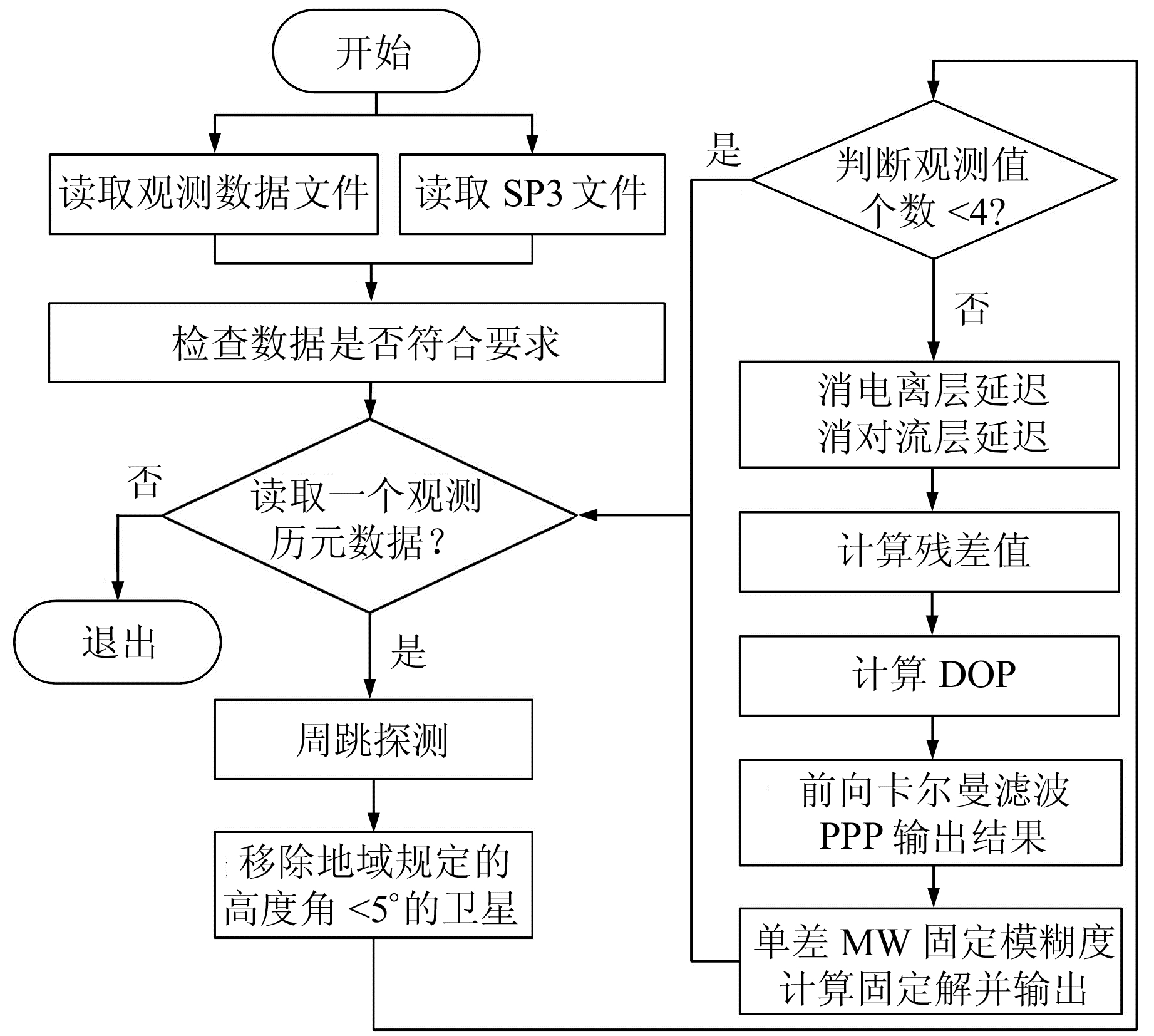图 1 PPP数据处理流程 Fig. 1 PPP data processing flow chart
3 实验结果及分析

3.1 可视卫星数及DOP分析

GPS与GPS+Iridium的平均可视卫星数随时间的变化如图 2所示，GPS+Iridium与GPS相比，每个历元平均增加1.5颗可视卫星。GPS与GPS+Iridium的GDOP、PDOP、HDOP、VDOP的平均值随时间的变化如图 3所示，由图可知，GPS+Iridium与GPS相比，每个历元的GDOP平均值从2.17降至1.9，平均降低0.27；PDOP平均值从1.9降至1.68，平均降低0.22；每个历元的HDOP平均值从1.04降至0.92，平均降低0.12；每个历元的VDOP平均值从1.58降至1.40，平均降低0.18。当Iridium星座增强GPS时，增加了可视卫星数，降低了精度因子，与水平精度因子相比，垂直精度因子降低的效果更为明显。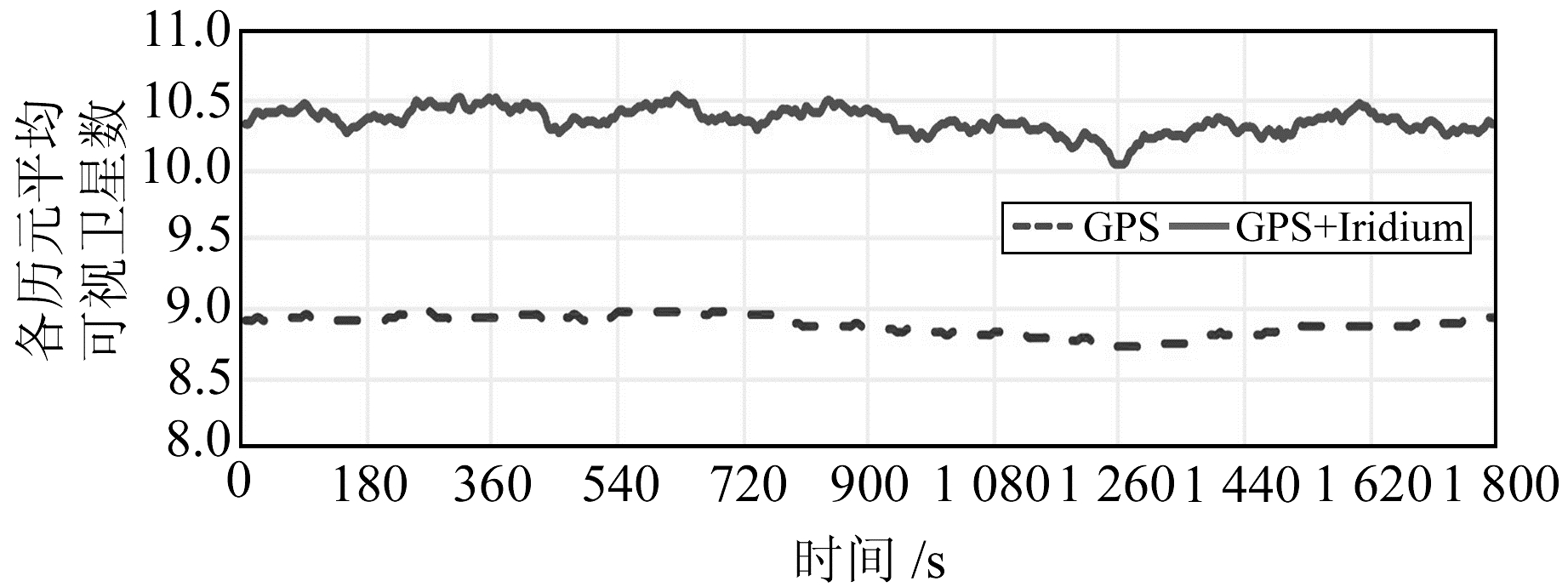图 2 GPS与GPS+Iridium的平均可视卫星数随时间的变化 Fig. 2 The visual satellite averages of GPS and GPS+Iridium change over time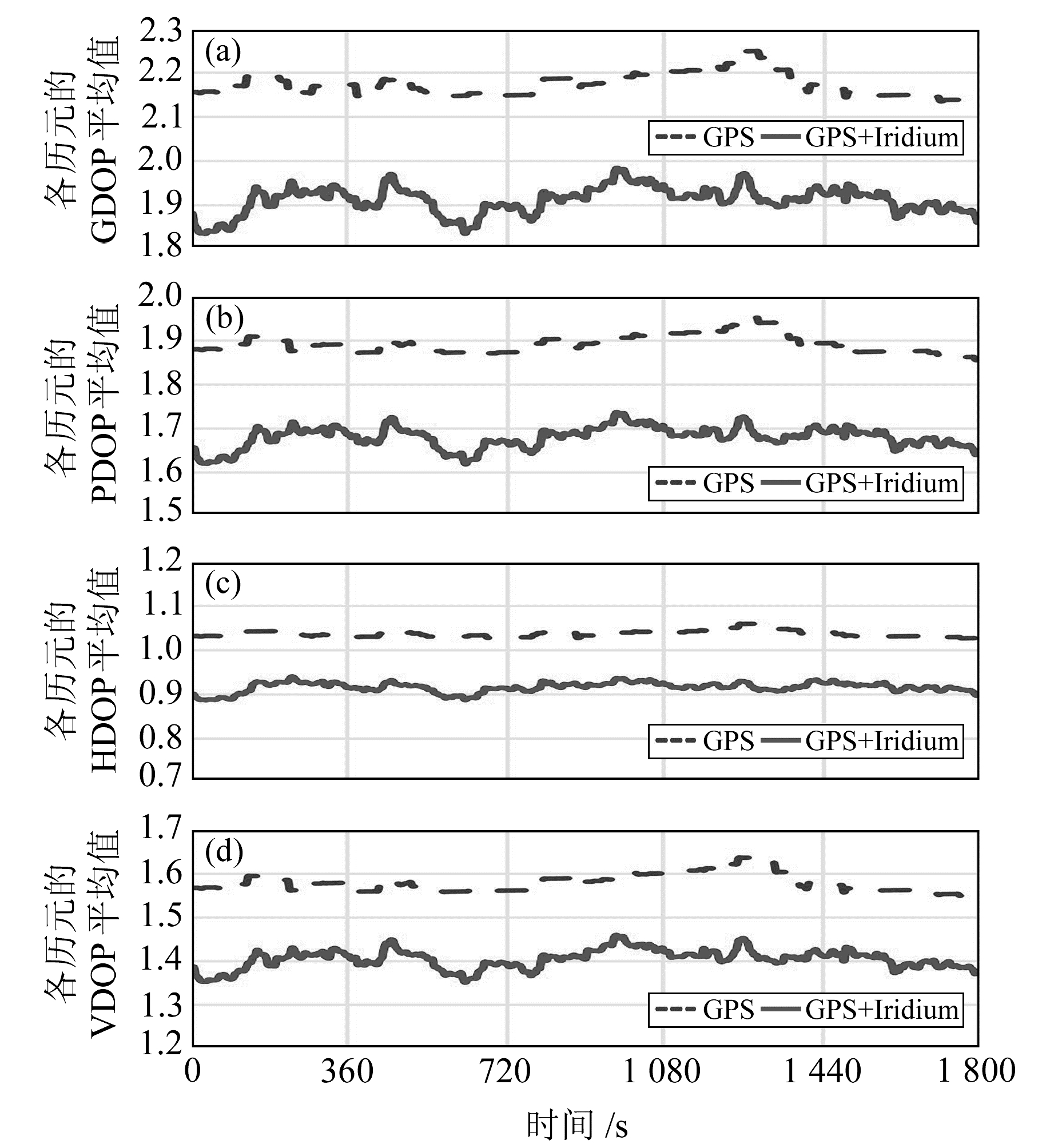图 3 GPS与GPS+Iridium的GDOP、PDOP、HDOP、VDOP的平均值随时间的变化 Fig. 3 GDOP, PDOP, HDOP and VDOP values of GPS and GPS+Iridium change over time
3.2 PPP滤波矩阵的病态性

 $\left\{ {\begin{array}{*{20}{c}} {{\mathit{\boldsymbol{X}}_{k + 1}} = {\mathit{\boldsymbol{ \boldsymbol{\varPhi} }}_{k + 1, k}}{\mathit{\boldsymbol{X}}_k} + {\mathit{\boldsymbol{ \boldsymbol{\varOmega} }}_k}}\\ {{\mathit{\boldsymbol{L}}_{k + 1}} = {\mathit{\boldsymbol{B}}_{k + 1}}{\mathit{\boldsymbol{X}}_{k + 1}} + {\mathit{\boldsymbol{ \boldsymbol{\varDelta} }}_{k + 1}}} \end{array}} \right.$ (1)

1) 预测：

 ${\mathit{\boldsymbol{X}}_{k + 1, k}} = {{\rm{\Phi }}_{k + 1, k}}{\mathit{\boldsymbol{X}}_k}$ (2)
 ${\mathit{\boldsymbol{D}}_X}_{_{k + 1, k}} = {\mathit{\boldsymbol{ \boldsymbol{\varPhi} }}_{k + 1, k}}{\mathit{\boldsymbol{D}}_X}_{_k}\mathit{\boldsymbol{ \boldsymbol{\varPhi} }}_{k + 1, k}^{\rm{T}} + {\mathit{\boldsymbol{D}}_{\rm{\varOmega }}}_{_k}$ (3)

2) 修正：

 ${\mathit{\boldsymbol{D}}_X}_{_{k + 1}} = {\left( {\mathit{\boldsymbol{D}}_{{X_{k + 1, k}}}^{ - 1} + \mathit{\boldsymbol{B}}_{k + 1}^{\rm{T}}\mathit{\boldsymbol{D}}_{{\Delta _{k + 1}}}^{ - 1}{\mathit{\boldsymbol{B}}_{k + 1}}} \right)^{ - 1}}$ (4)
 ${\mathit{\boldsymbol{X}}_{k + 1}} = {\mathit{\boldsymbol{D}}_{{X_{k + 1}}}}(\mathit{\boldsymbol{D}}_{{X_{k + 1,k}}}^{ - 1}{\mathit{\boldsymbol{X}}_{k + 1,k}} + \mathit{\boldsymbol{B}}_{k + 1}^{\rm{T}}\mathit{\boldsymbol{D}}_{{\Delta _{k + 1}}}^{ - 1}{\mathit{\boldsymbol{L}}_{k + 1}})$ (5)

 ${\rm{cond}}\left( \mathit{\boldsymbol{N}} \right) = \left\| {{\mathit{\boldsymbol{N}}^{ - 1}}} \right\| \cdot \left\| \mathit{\boldsymbol{N}} \right\| = \frac{{{\lambda _{{\rm{max}}}}}}{{{\lambda _{{\rm{min}}}}}}$ (6)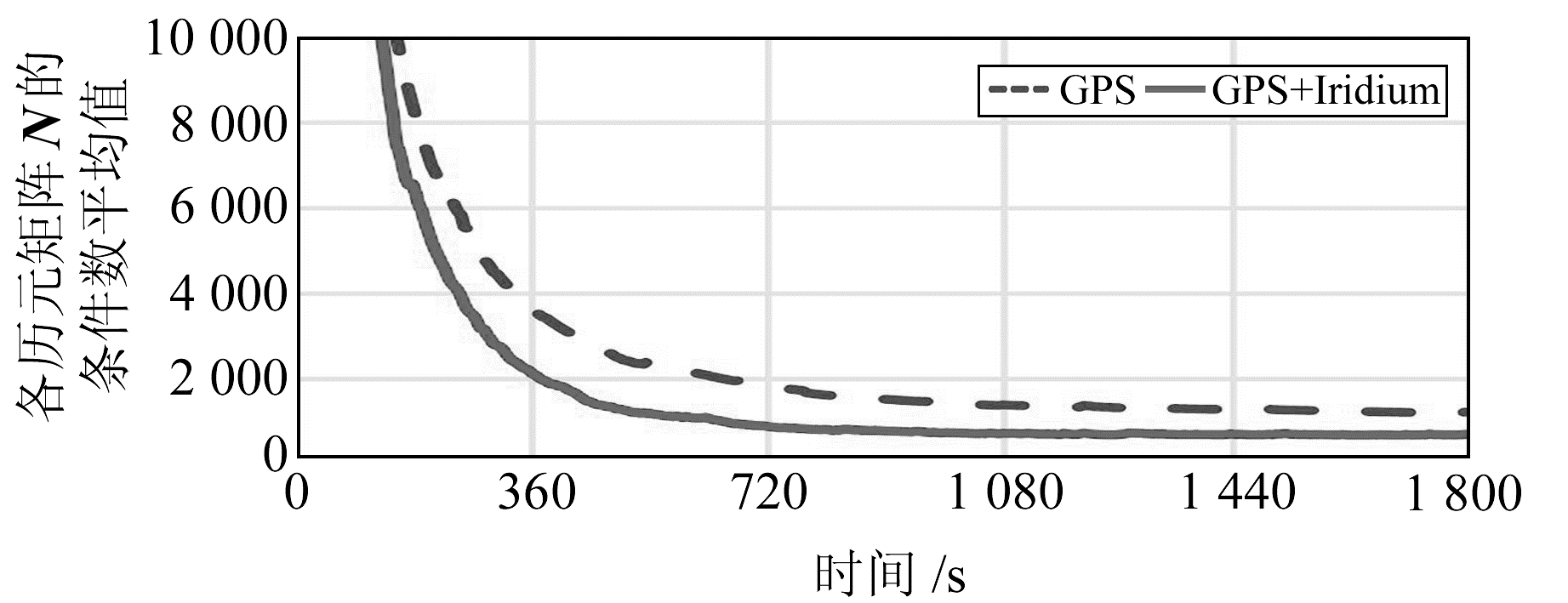图 4 GPS与GPS+Iridium滤波矩阵N的条件数平均值随时间的变化 Fig. 4 The mean value of the conditional number of the filtration matrix N of GPS and GPS+Iridium change over time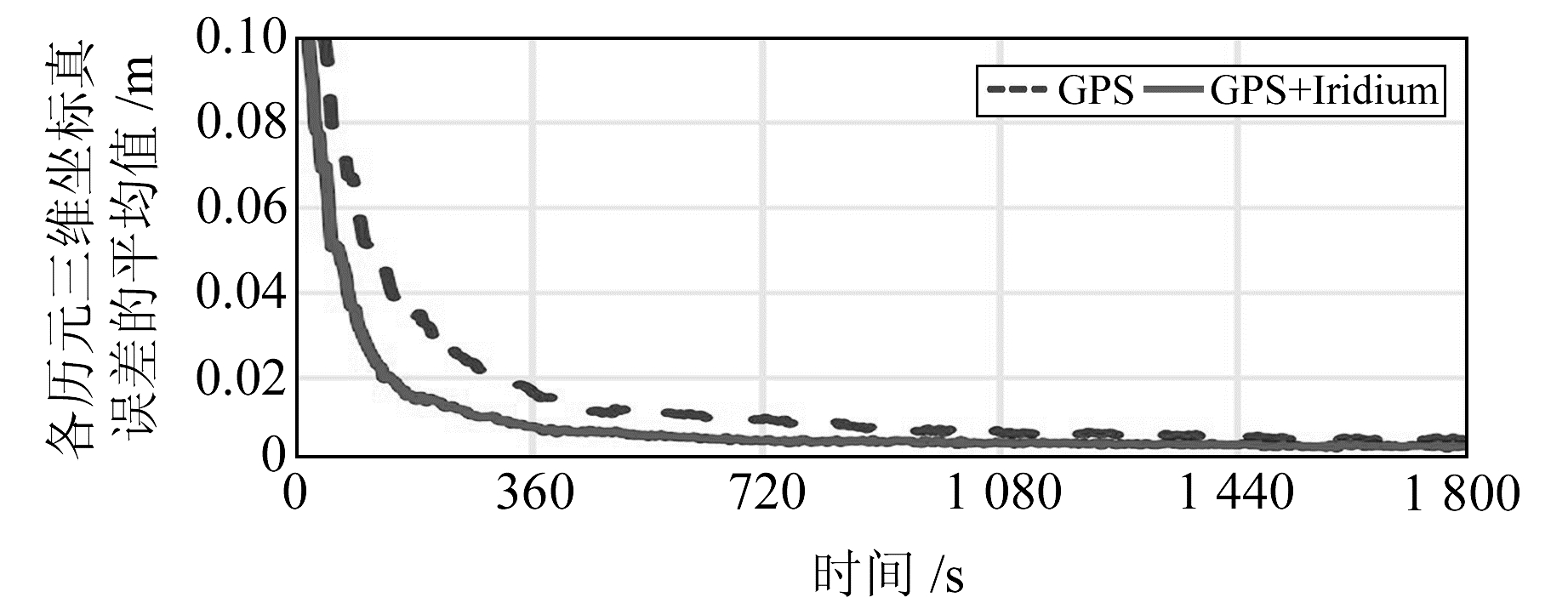图 5 GPS与GPS+Iridium三维坐标真误差的平均值随时间的变化 Fig. 5 The mean value of three-dimensional coordinate true error of GPS and GPS+Iridium change over time
3.3 PPP收敛时间及精度分析

PPP收敛时间过长则无法快速定位，影响PPP的作业效率。PPP收敛时间受观测数据质量、卫星轨道与钟差、测站纬度、电离层与对流层等误差模型、待估参数个数、卫星空间几何构型等因素的影响，其中对流层模型和卫星空间几何构型GDOP对PPP收敛时间的影响非常明显。GPS+Iridium双系统与GPS单系统相比，在其他因素不变的情况下，Iridium星座使卫星空间几何构型发生了重大变化。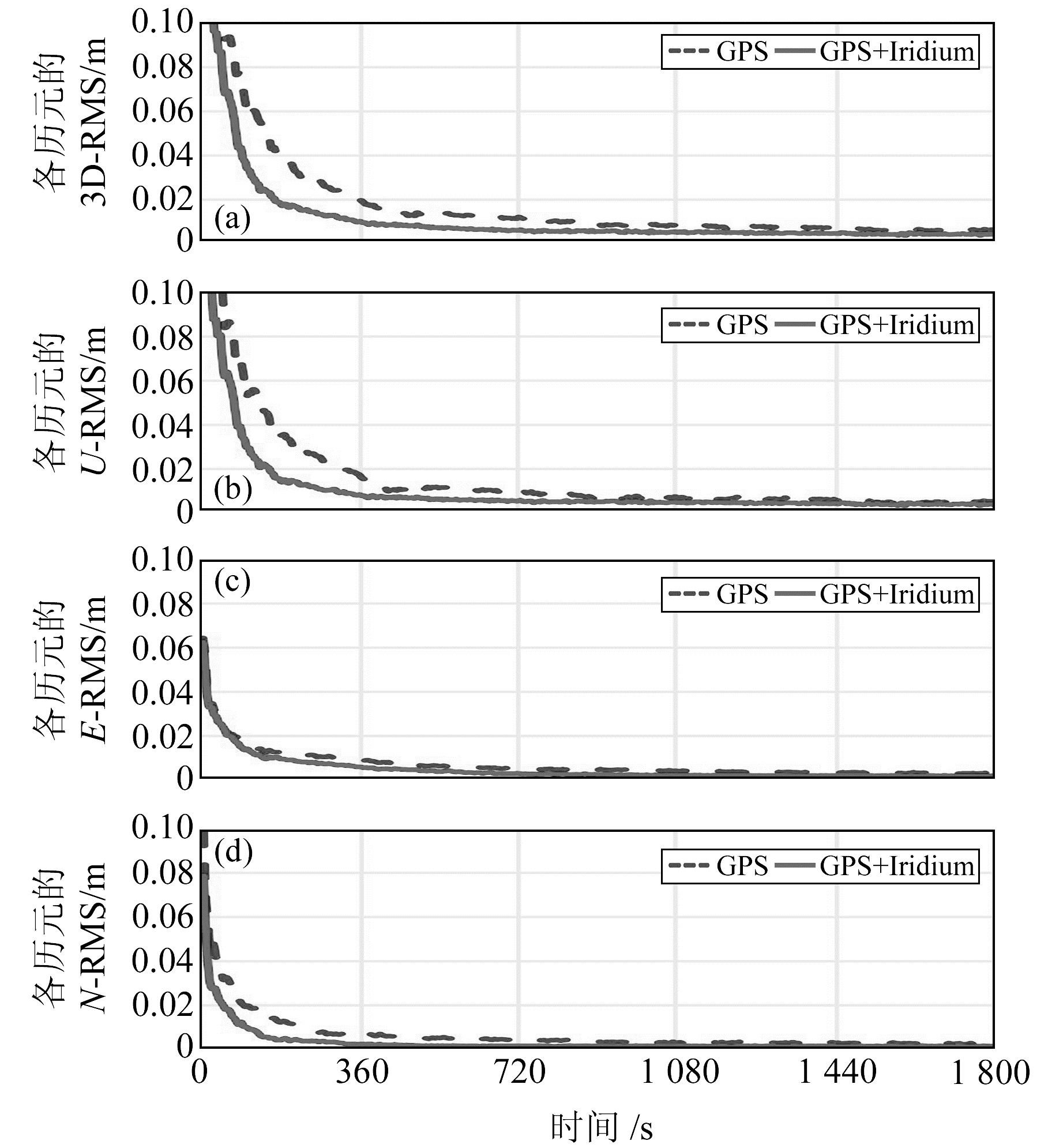图 6 GPS与GPS+Iridium定位结果随时间的变化 Fig. 6 The positioning results of GPS and GPS+Iridium changes over time

PPP处理结果中，GPS单系统的3D-RMS在55 s时小于0.1 m，在145 s时小于0.05 m，在245 s时小于0.03 m，最终收敛在0.006 m左右；GPS+Iridium双系统的3D-RMS在30 s时小于0.1 m，在80 s时小于0.05 m，在120 s时小于0.03 m，最终收敛在0.004 m左右，与GPS相比收敛时间分别缩短了45%、45%、51%，收敛后精度提高了33%。GPS单系统的U-RMS在140 s时小于0.05 m，在210 s时小于0.03 m，在425 s时小于0.01 m；GPS+Iridium双系统的U-RMS在80 s时小于0.05 m，在105 s时小于0.03 m，在280 s时小于0.01 m，与GPS相比收敛时间分别缩短了43%、50%、34%。对于N方向，在收敛前GPS+Iridium双系统的RMS比GPS单系统降低了0.01 m左右。

3.4 PPP模糊度首次固定成功的时间表 1 GPS+Iridium与GPS的模糊度首次固定成功所需时间的统计 Tab. 1 The statistics of the time required for the first fixed success of ambiguity of GPS+Iridium and GPS
4 结语

  邓琳, 李广侠, 田世伟, 等. 基于LEO增强的COMPASS导航系统抗干扰能力研究[J]. 军事通信技术, 2012, 33(2): 65-59 (Deng Lin, Li Guangxia, Tian Shiwei, et al. Capabilities of LEO Enhanced COMPASS Navigation System for Anti-jamming[J]. Journal of Military Communications Technology, 2012, 33(2): 65-59) (0)  Tian S W, Dai W H, Liu R F, et al. System Using Hybrid LEO-GPS Satellites for Rapid Resolution of Integer Cycle Ambiguities[J]. IEEE Transactions on Aerospace and Electronic Systems, 2014, 50(3): 1 774-1 785 DOI:10.1109/TAES.2014.120662 (0)  赵金峰, 于笑, 冯少栋, 等. 基于LEO增强的COMPASS系统设计及性能分析[J]. 电讯技术, 2013, 53(2): 131-135 (Zhao Jinfeng, Yu Xiao, Feng Shaodong, et al. Design and Performance Analysis of LEO Satellites Enhanced COMPASS System[J]. Telecommunication Engineering, 2013, 53(2): 131-135) (0)  俞能杰, 方晖, 王盾, 等.基于低轨星座的GNSS信号全球监测约束条件初步分析[C].第六届中国卫星导航学术年会, 西安, 2015 (Yu Nengjie, Fang Hui, Wang Dun, et al. The Preliminary Constraints Analysis of GNSS Signals Global Monitoring Based on LEO Constellation[C]. The Sixth China Satellite Navigation Conference, Xi'an, 2015) https://kns.cnki.net/KCMS/detail/detail.aspx?dbcode=IPFD&filename=WXDH201505005007 (0)  王亚菲, 钟世明, 王海涛, 等. LEO卫星轨道预报精度分析[J]. 测绘学报, 2016, 45(9): 1 035-1 041 (Wang Yafei, Zhong Shiming, Wang Haitao, et al. Precision Analysis of LEO Satellite Orbit Prediction[J]. Acta Geodaetica et Cartographica Sinica, 2016, 45(9): 1 035-1 041) (0)  方善传, 杜兰, 周佩元, 等. 低轨导航增强卫星的轨道状态型星历参数设计[J]. 测绘学报, 2016, 45(8): 904-910 (Fang Shanchuan, Du Lan, Zhou Peiyuan, et al. Oribital List Ephemerides Design of LEO Navigation Augmentation Satellite[J]. Acta Geodaetica et Cartographica Sinica, 2016, 45(8): 904-910) (0)  Pratt J, Axelrad P, Larson K M, et al. Satellite Clock Bias Estimation for iGPS[J]. GPS Solutions, 2013, 17(3): 381-389 DOI:10.1007/s10291-012-0286-4 (0)  Fossa C E, Raines R A, Gunsch G H, et al. An Overview of the IRIDIUM(R) Low Earth Orbit(LEO) Satellite System[C]. Proceeding of the IEEE 1998 National Aerospace and Electronics Conference, Dayton, 1998 https://www.researchgate.net/publication/3766499_An_Overview_of_the_Iridium_Low_Earth_Orbit_LEO_Satellite_System (0)  Salazar D, Hernandez-Pajares M, Juan J M, et al. GNSS Data Management and Processing with the GPSTk[J]. GPS Solutions, 2009, 14(3): 293-299 (0)  李建文, 李作虎, 周巍, 等. 卫星导航中几何精度衰减因子最小值分析及应用[J]. 测绘学报, 2011, 40(增1): 85-88 (Li Jianwen, Li Zuohu, Zhou Wei, et al. Study on the Minimum of GDOP in Satellite Navigation and Its Applications[J]. Acta Geodaetica et Cartographica Sinica, 2011, 40(S1): 85-88) (0)  曹相, 高成发. GPS精密单点定位(静态)影响收敛速度的因素分析[J]. 现代测绘, 2007, 30(1): 19-21 (Cao Xiang, Gao Chengfa. Factors Analyze of the Convergence Rate for GPS Precise Point Positioning[J]. Modern Surveying and Mapping, 2007, 30(1): 19-21 DOI:10.3969/j.issn.1672-4097.2007.01.006) (0)  赵兴旺, 王胜利, 刘超. GNSS精密单点定位理论与方法[M]. 合肥: 中国科学技术大学出版社, 2015 (Zhao Xingwang, Wang Shengli, Liu Chao. Theory and Method of GNSS Precise Point Positioning[M]. Hefei: University of Science and Technology of China Press, 2015) (0)
Precise Point Positioning Primary Analysis of GNSS Enhanced with LEO Constellation
ZHAO Xinglong1,2,3ZHONG Shiming2     OU Jikun2     ZHOU Chongchong2,3     TANG Chengpan1
1. Shanghai Astronomical Observatory, CAS, 80 Nandan Road, Shanghai 200030, China;
2. Institute of Geodesy and Geophysics, CAS, 340 Xudong Street, Wuhan 430077, China;
3. University of Chinese Academy of Science, A19 Yuquan Road, Beijing 100049, China
Abstract: This paper analyzes the LEO constellation's enhancement effect on GNSS's precise point positioning(PPP), adopting mathematical simulation technology and taking the GPS system and the Iridium system as examples. Based on the emulational observation data of the GPS and Iridium, taking BJFS station as an example, pseudo- kinematic precise point positioning experiment is performed. The test results show that, compared with the GPS single system, the number of visible satellites of the GPS+Iridium dual system increases by an average of 1.5. The GDOP value and the PDOP value decrease by about 0.25. With Iridium enhanced GPS, the condition number of PPP filtration matrix reduces rapidly and the stability of PPP filtration is promoted; the positioning accuracy of the zenith U-direction, along with the three-dimensional positioning accuracy; the PPP convergence time is shortened by 30% or more and single-difference MW method is adopted to fix the PPP ambiguity. With Ratio =1.5, 3, 5 as an index of ambiguity fixed successfully, and compared with the emulational value of ambiguity, the time required for the first successful fixing of PPP ambiguity is shortened by 40% or more.
Key words: LEO satellite; GNSS; enhancement; PPP